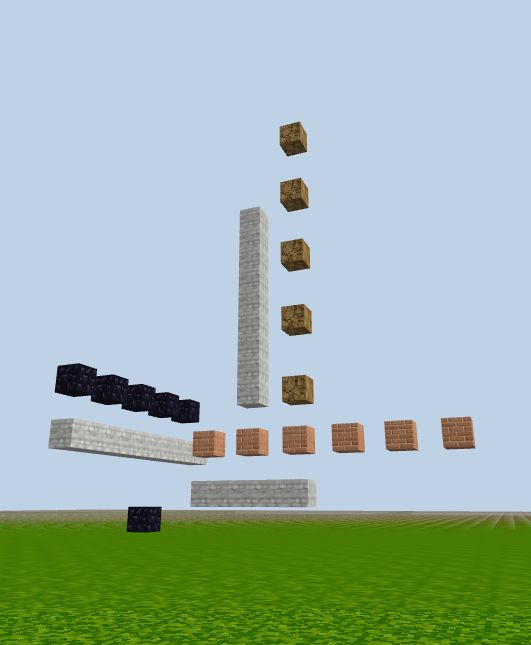## Solid Line & Dotted Line

By alternating the starting/ending value of the loop counter or the increment step, we can design solid lines at any location or dotted lines with different steps.

Solid lines that don't start at the origin:

``````for (let i = 3; i < 8; i++) {
game.setBlock(new Position(i, 2, -20), 18);
}

for (let j = 6; j < 15; j++) {
game.setBlock(new Position(5, j, -20), 18);
}

for (let k = 15; k < 25; k++) {
game.setBlock(new Position(0, 4, -k), 18);
}
``````

Dotted lines with different steps:

``````for (let i = 3; i < 15; i += 2) {
game.setBlock(new Position(i, 4, -20), 17);
}

for (let j = 6; j < 20; j += 3) {
game.setBlock(new Position(7, j, -20), 20);
}

for (let k = 15; k < 25; k += 2) {
game.setBlock(new Position(0, 6, -k), 67);
}
``````